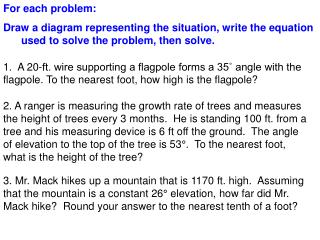DownloadDownload PresentationFor each problem:

# For each problem:

Download Presentation## For each problem:

- - - - - - - - - - - - - - - - - - - - - - - - - - - E N D - - - - - - - - - - - - - - - - - - - - - - - - - - -
##### Presentation Transcript

1. For each problem: Draw a diagram representing the situation, write the equation used to solve the problem, then solve. 1. A 20-ft. wire supporting a flagpole forms a 35˚ angle with the flagpole. To the nearest foot, how high is the flagpole? 2. A ranger is measuring the growth rate of trees and measures the height of trees every 3 months. He is standing 100 ft. from a tree and his measuring device is 6 ft off the ground. The angle of elevation to the top of the tree is 53°. To the nearest foot, what is the height of the tree? 3. Mr. Mack hikes up a mountain that is 1170 ft. high. Assuming that the mountain is a constant 26° elevation, how far did Mr. Mack hike? Round your answer to the nearest tenth of a foot?

2. 8.3 Solving Right Triangles Objective Use trigonometric ratios to find angle measures in right triangles and to solve real-world problems.

3. 8.3 Solving Right Triangles • 1. What is the sin of 30° • What is the sin of A of the triangle. • What do you think the measure of B is? 8 in 20 in 4 in 10 in A B

4. 8.3 Solving Right Triangles If you know the angle measure, then you can calculate the sin, cos or tan of that angle (that is the ratio of the sides). If you know the side lengths of a right triangle, then you can also determine the angle measure. Previously we’ve solved problems where you were given the angle measure and you found the side lengths. Now you’ll be given the ratio of the side lengths and you’ll determine the angle measure.

5. 8.3 Solving Right Triangles For example, what is the angle measure of angle A? We need to find the angle measure whose sin = 14/25 or 0.56 25 in 14 in A Use the given trigonometric ratio to determine which angle of the triangle is A. 17 8 cos Z = 8/17 15 tan b = 8/15

6. 8.3 Solving Right Triangles What angle has a… cos = 0.50 cos = 0.7 cos = 0.12 sin = 0.6 sin = 0.25 sin = 0.8 tan = 0.6 tan = 1.25 tan = 1

7. 8.3 Solving Right Triangles A 22 42 30 26 12 A A 12

8. HOMEWORK 8.3 (555):21-35,39-44,47